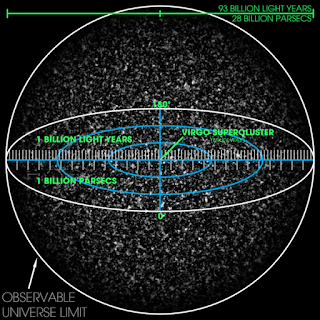## Wednesday, 22 April 2020

### Exercise 5.3 Inside the event horizon

Question
Consider a particle (not necessarily on a geodesic) that has fallen inside the event horizon, $r<2GM$. Use the ordinary Schwarzschild coordinates $\left\{t,r,\theta,\phi\right\}$.Observable Universe

a) Show that the radial coordinate must decrease at a minimum rate given by $$\left|\frac{dr}{d\tau}\right|\geq\sqrt{\frac{2GM}{r}-1}$$b) Calculate the maximum lifetime for a particle along a trajectory from $r=2GM$ to $r=0$.
c) Express this in seconds for a black hole with mass measured in solar masses.
d) Show that this maximum proper time is achieved by falling freely with $E\rightarrow0$.
The most interesting exercise so far, especially parts b and c. If the sun were a black hole then the maximum lifetime for our particle would be $${\Delta\tau}_\bigodot=1.55\times\ {10}^{-5}\ \rm{s}$$That's a pretty short time, but if the Sun were a black hole it would have $2GM=3\ \text{km}$ so our test particle would have an average speed of about $2\ \times\ {10}^8\ \text{m s}^{-1}$ which is just below the speed of light and a hundred times faster than the Parker Solar Probe launched in 2018 which should only reach 0.064% the speed of light.
However big the black hole is, the average minimum speed for the fall for the centre is constant at$$v_\rm{AvMin}=\frac{2c}{\pi}$$M87* the black hole at the centre of our galaxy is about $6.5\times\ {10}^9$ solar masses so we get$${\Delta\tau}_\rm{M87\ast}=1.55\times\ {10}^{-5}\times6.5\times\ {10}^9={10}^5\ s=28\ \rm{hours}$$There is a short time to prepare in M87*.
We can do the same for a black hole with the mass of the Universe: The observable Universe contains ordinary matter equivalent to ${10}^{23}$ solar masses. So$${\Delta\tau}_{Universe}=1.55\times\ {10}^{-5}\times{10}^{23}\approx{10}^{18}s=300\ \text{billion years}$$The estimated 'age' of the Universe is 14 billion years, so there is plenty of time inside the big black hole - we have hardly started the journey.